# The Progression of Fractions

## Exploring Fraction Constructs and Proportional Reasoning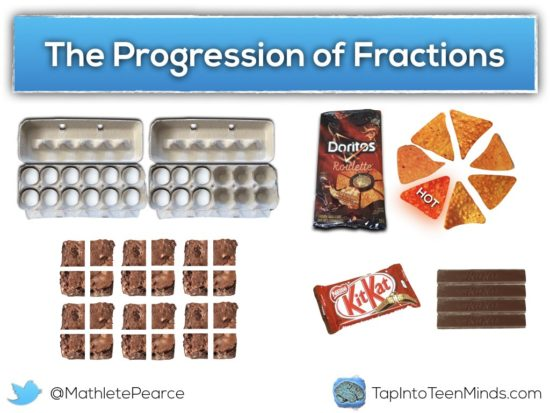Fractions are a beast of a concept that causes struggles for many adults and students alike. While we all come to school with some intuition to help us with thinking fractionally and proportionally, the complexity quickly begins to increase as we move from concrete, to visual, to symbolic and from identifying, to comparing, to manipulating. Fractions are formally introduced in the Ontario Math Curriculum when students begin dividing whole objects into pieces and identify these pieces using fractional names (e.g.: halves; fourths or quarters) and continues to promote the development fractional fluency concretely through each grade. Interestingly enough, it is often said that students struggle much more with numbers represented in fraction notation than those represented in decimal notation, yet the word “fraction” appears in the curriculum document 99 times beginning in grade 1, while the word “decimal” appears only 69 times beginning three years later in the 4th grade. While it might be true that fractions tend to intimidate, I wonder if our dependence on the calculator has tricked us into believing we are more fluent with quantities represented in decimal form than is reality.

## Paying Attention to Fractions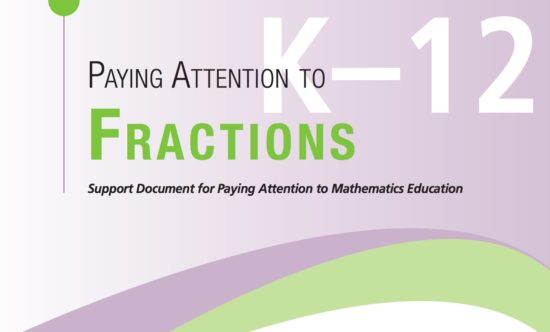As I did with the Progression of Proportional Reasoning, I’d like to reference the Paying Attention To Mathematics series released by the Ontario Ministry of Education Literacy and Numeracy Secretariat (LNS) called Paying Attention to Fractions. These guides are a great start to help you wrap your head around big ideas in mathematics and thus, this post will attempt to expand on the ideas shared in this particular document.

## Why Fractions?

As if the struggles our students experience when working with fractions aren’t enough justification, I like this quote shared in the guide:

“No area of elementary school mathematics is as mathematically rich, cognitively complicated, and difficult to teach as fractions, ratios, and proportionality. These ideas all express mathematical relationships: fractions and ratios are ‘relational’ numbers. They are the first place in which students encounter numerals like ‘ 3/4 ’ that represent relationships between two discrete or continuous quantities, rather than a single discrete (‘three apples’) or continuous quantity (‘4 inches of rope’).”

(Litwiller & Bright, 2002, p. 3)

## What Is A Fraction?

A fraction is a number.

While fractional notation is typically used to represent quantities that are not whole, it is possible for all quantities to be represented as a fraction.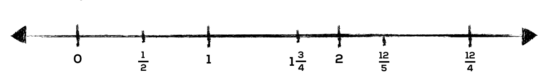While these descriptions are simple on the surface, they do not appropriately communicate the complex constructs that lie within this big idea.

## Connecting Proportional Reasoning and Fractions

If you recall from my last post, proportional reasoning is takes place when one compares two numbers in relative terms rather than absolute terms. Thus, it can be said that a fraction provides a means for representing that relationship between two numbers.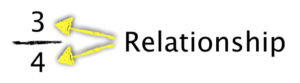It is reasonable to believe that young learners begin working towards proportional reasoning even before they enter school when they make comparisons between two quantities such as:

• this bag is heavier than that one;
• I’m “this much” shorter than you are; and,
• there are 5 more red candies than green.

While these early comparisons are likely absolute (i.e.: additive) in nature, we are one step closer to comparing these quantities relatively (i.e.: multiplicatively).

Let’s take some time to explore four common fraction constructs.

## Counting Cartons: Part-Whole Relationships

Have a look at the image below: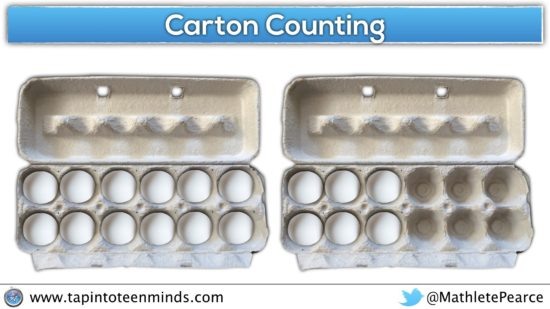I might ask students to determine how many eggs there are. This question is quite clear that I would like to know the quantity of eggs, however they get to decide how they would like to represent that quantity. While many may simply choose to represent the number of eggs as 18, others may get creative and unitize (i.e.: 3 groups of 6; 9 groups of 2; etc.) or even reference the number of eggs relative to egg cartons.

After students share out with a partner, I might ask students:

how many cartons of eggs are there?

After students have some time to think independently, I would have them share out with their table groups. Some interesting responses are sure to come up such as:

• 18/2
• 18/1.5
• 3/2
• 1.5
• 2

You may notice that by asking students to state how many cartons of eggs there are, there is room for interpretation. Some students might simply state that there are two egg cartons without considering the number of eggs present, some might give a quantity relative to two egg cartons, while others might state the number of eggs relative to a single carton.

After students have been given an opportunity to defend their thinking, I might ask students to state how many cartons are full of eggs?

This fraction construct would be an example of a part-whole relationship. Students might consider how many whole and partial cartons there are in the form of a mixed fraction (1 and 1/2), improper fraction (3/2) or in decimal form (1.5). Some common representations for these different fractional forms might be using a set model, area model or number line. The following animated gif shows each of these representations, sometimes more than one simultaneously: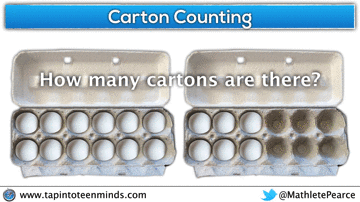You might have noticed the double-number line included in the animated gif. This idea can be very useful when making the jump from thinking fractionally to proportional reasoning.

## Doritos Roulette: Part-Part Relationships

In the Doritos Roulette 3 Act Math Task, students are asked questions that stem from the relationship of “hot” chips to “not hot” chips based on the image on the front of the bag: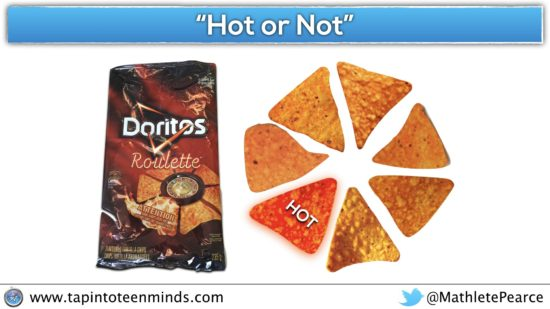I might ask students to consider the image above and ask them this question:

What fraction of hot chips to not hot chips are there in a bag of Doritos Roulette?

Here are some common responses I see when I ask students (and teachers) this question:

• 1/6
• 1/7
• 1:6
• 1:7

Despite the explicit request for a part-part relationship between “hot” chips (1) and “not hot” chips (6), we often see the relationship between “hot” chips (1) and the whole (7).

Something I might ask the group to discuss with their neighbours is whether we are “allowed” to represent a part-part relationship as a fraction. Great (and sometimes heated) discussions can arise from this question, which makes this exciting to facilitate.

The discourse this question can promote is a great way to address the fact that part-part relationships are commonly represented as a ratio, but that both part-part relationships and ratios can also be represented as a fraction. In this case, the numerator represents the number of “hot” chips; the denominator represents the number of “not hot” chips; and the sum of each part, the numerator and denominator, is the whole: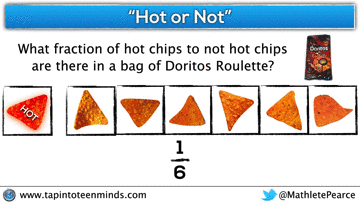After exploring part-whole and part-part fraction constructs, you may begin to notice how important clarity around what a given fraction represents prior to attempting any comparison or manipulation of that fraction.

While the use of a set model and area model remain fairly similar regardless of whether you are dealing with a part-whole or part-part relationship, the use of a number line changes significantly.

Recall how the number line used in the part-whole counting cartons example made identifying the part and the whole fairly intuitive: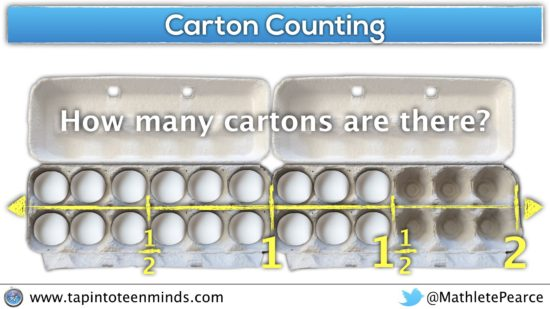When we attempt to use a number line with a part-part relationship, it becomes more difficult to determine the size of the second part without modifying how we use this representation: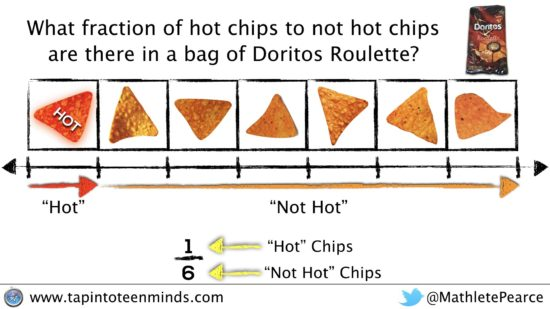Note that in the representation above, two parts are clearly shown and the sum of those two parts represents the whole.

Although the number line representation might not be as intuitive in the part-part case, it does help you visualize both parts proportionally: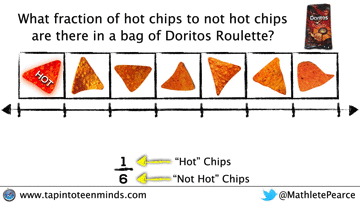Extending from a single number line to a double-number line can help shine a light on the meaning behind the part-part relationship. In this case, there are one-sixth as many “hot” chips as there are “not hot” chips. Alternatively, there are 6 times as many “not hot” chips as there are “hot” chips.

## Baking Brownies: Fraction as Quotient

When considering fractions as quotient, we are referring to the times when we are dividing two numbers. The Paying Attention to Fractions document uses an example of kids fair sharing some brownies. Let’s keep the context the same, but I’ll add some visuals to enhance the experience.

Suppose there are 6 brownies that are to be shared amongst 4 friends fairly. How much should each person receive?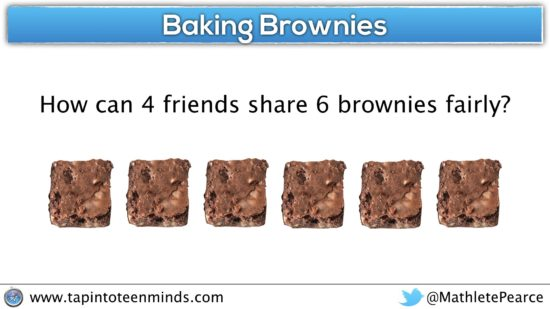Like many situations in life, there are multiple ways to share these brownies fairly. Let’s represent a few of these methods.

### Partitioning Brownies into Fourths

Since there are 4 people to share the 6 brownies, we can divide the 6 brownies into fourths and then pass them out, one-by-one: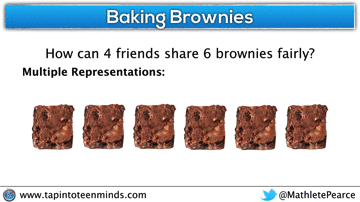After splitting the brownies into quarters, you’ll notice that each of the four people should receive 6 quarters. Hence why we can represent this fraction as quotient as the improper fraction, 6/4.

### Sharing Full Brownies Equally, Then Partitioning Remainder

Some people might find it useful to share whole brownies equally and then when there are not enough whole brownies to share fairly, consider partitioning up the remaining brownies appropriately: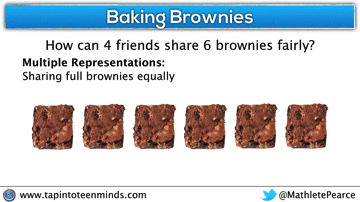In the case with 6 brownies, we can share one brownie each and then partition the remaining brownies into four halves to share. This method would be equivalent to the mixed fraction of 1 and 1/2 brownies per person.

### Partition Brownies Into Four Equal Portions

This method involves considering all 6 brownies and where cuts must be made to create four equal groupings: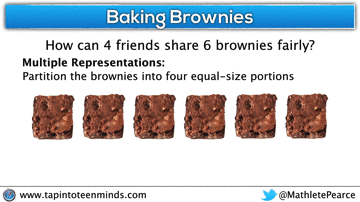This method would be another representation of the mixed fraction, 1 and 1/2 brownies for each person.

For each of the methods above, we could also consider the use of a number line to support the partitioning of the brownies to distribute a fair amount to each person. Here are a few examples: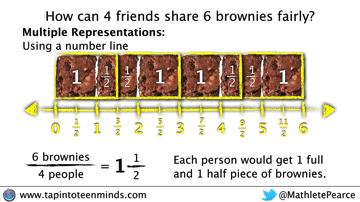## How Many Rolos: Fraction as Operator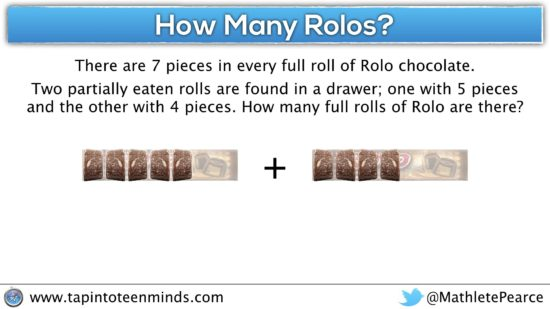Another common construct is the fraction as operator.

Let’s have a look at another problem. We mind as well keep the context as food, because that is just yummy.

There are 7 pieces in every full roll of Rolo chocolate.

Two partially eaten rolls are found in a drawer; one with 5 pieces and the other with 4 pieces.

How many full rolls of Rolo are there?

If we use concrete manipulatives or visuals, like we will in this instance, it is much easier to see how to add and subtract fractions. In this case, we’ll show a few different representations including how using a number line can be helpful: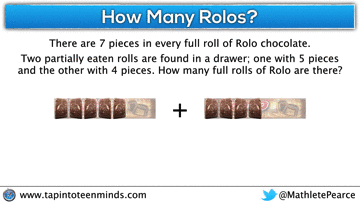So in this particular example, you can see that 5 pieces plus 4 pieces will yield 9 pieces, which is more than a full 7 piece roll; 9/7 or 1 and 2/7.

## Fraction As Operator: Multiplication

If you’re a 3 act math fan, you might be familiar with my Gimme a Break 3 act math task involving the use of fractions as operators. I should note that while there are some useful pieces in that task, it has really evolved over time and I just haven’t had the time to update the post yet. Here’s some of the pieces I’ve added along the way.

The task begins with an opportunity for students to notice/wonder after watching a video of me opening a KitKat bar.

Later in the task, the following video is shown asking students to consider what operation could be taking place:

Since the video is open ended, I’m always hoping that all students will be able to share something mathematical about the situation. For example, we might see some responses like these: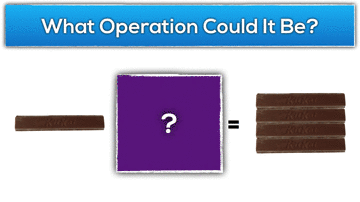However, the new learning I’m hoping to spend some time on is the multiplication of a fraction by a whole number. In this case, a student might think of this situation as 4 groups of one quarter of a whole bar: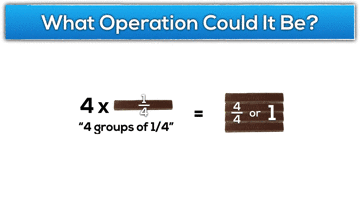Alternatively, we could think of this situation as one quarter groups of four whole KitKat bars: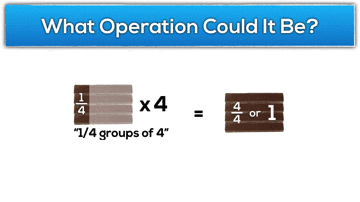In either case, the use of arrays and/or area models can be very useful when trying to help build an understanding of multiplication with fractions. In the following example, we extend this idea to the multiplication of two fractions: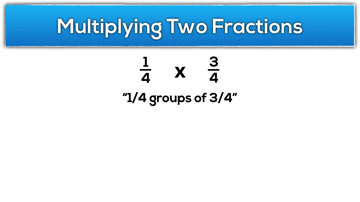While I find that multiplication of fractions seems to be the one operator I hear the least noise about, my gut tells me that it is more about being able to remember the algorithm and not due to any conceptual understanding. In my experience, many students can manage to remember to “multiply the tops, multiply the bottoms” without necessarily having any real conceptual understanding to fall back on in times of doubt.

## Fraction As Operator: Division

On the other hand, division of fractions seems to be a sore spots for many students (and adults) because the algorithm is a bit more complicated and less intuitive. Pair that with a very low level of conceptual understanding as to why the algorithm actually works and you’ll end up with confusion and frustration for many. The gap between the pure memorization of steps and the conceptual understanding of what dividing fractions really means is often so large that many educators simply follow suit and focus purely on the algorithm. While I believe it is so important to build the conceptual understanding behind a complex idea like dividing fractions, I know that the task can be quite difficult and many teachers aren’t so sure how to approach it. When we rush to an algorithm before constructing conceptual understanding, students (and adults) are often very difficult to engage in the heavy thinking required to connect the dots. This leaves many teachers believing that successful use of the algorithm is enough (or all they have time for) and they just move on to the next topic.

Let’s look at the Gimme a Break context in terms of division of fractions. With manipulatives on the table such as pattern blocks or relational rods, I might ask students:

What would 1 ÷ 1/4 “look like” in terms of the KitKat task?

Can you show a neighbour what that looks like to you?

Hopefully students come up with some representation to model what a whole KitKat bar would look like an a quarter of a KitKat bar: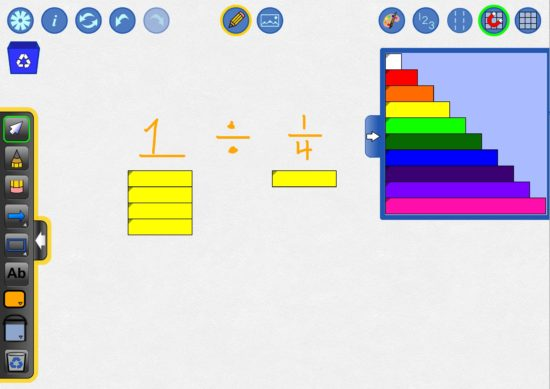Then, I would ask them to determine how many quarter pieces there are in a whole KitKat bar. With this in mind, students should be able to “see” and manipulate their representation on the table to come up with an answer of 4.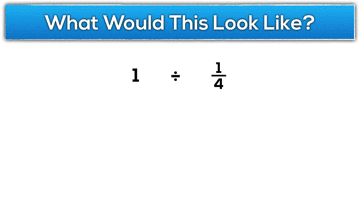Then, I might consider asking students to represent a couple more similar situations using whole numbers divided by a certain number of quarter pieces to build some confidence.

For example, 2 divided by 1/4: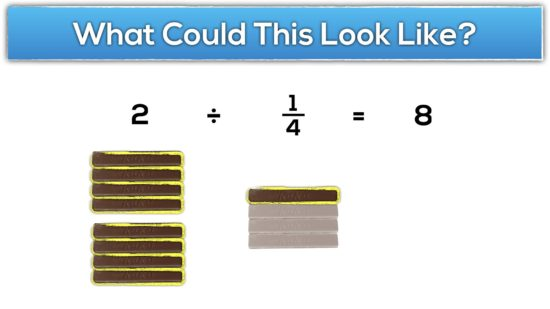3 divided by 3/4: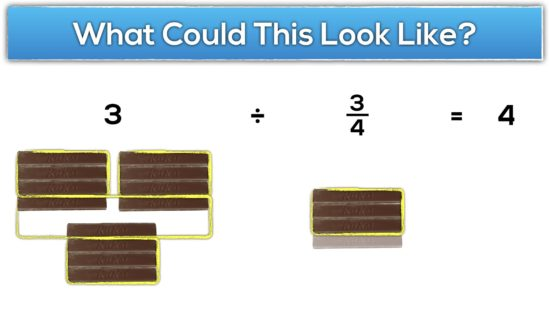When ready, students might be ready to start stepping outside of whole numbers to situations like 3/4 divided by 2/4: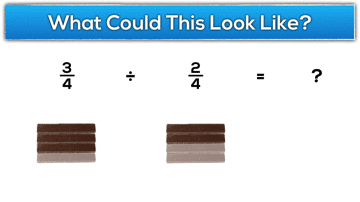Then, once students are really comfortable dividing fractions with common denominators, you might start moving to situations where the fractions have uncommon denominators.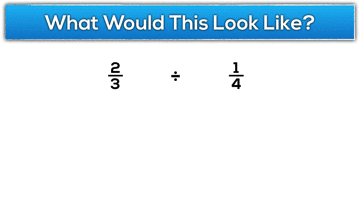Note that context is always very important for students to build a conceptual understanding. If we are trying to explain abstraction through abstraction (i.e.: explaining a rule algebraically based on another algebraic rule), then it is unlikely to stick. However, if we, the educators, spend the time necessary to understand mathematics conceptually, it will be a huge pay off for the understanding of our students. Only once students are able to visualize what mathematics “looks like” does it make sense for us to move towards more efficient methods like algorithms or more abstract problems where context is stripped away.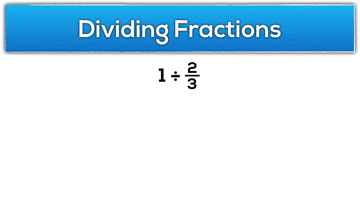Throughout this post we have tackled the idea of fraction constructs by using a similar approach to how I introduce most big ideas in math class: using tasks that are contextual, visual and concrete in order to build a conceptual understanding.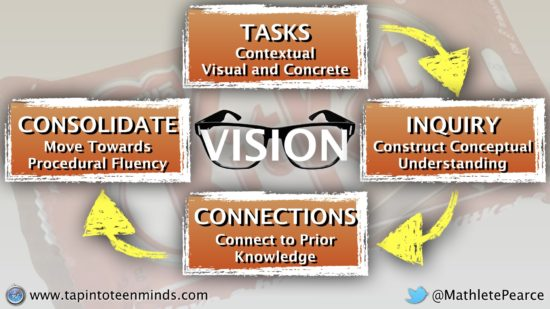This post is by no means the “fractions rulebook”, but rather a journal outlining my own journey to understanding fractions conceptually. As a secondary mathematics teacher, much of what our curriculum is built on is the assumed understanding of topics like fractions. However, the more I reflect on my own mathematical understanding, the more I realize that I never truly had a conceptual understanding of most concepts that I was teaching.

Let’s avoid the rush to the algorithm and slow down to let kids truly experience and understand mathematics. I’d appreciate any pieces I should add to this post in the comments section.

## Memorization vs. Automaticity

### What's The Difference?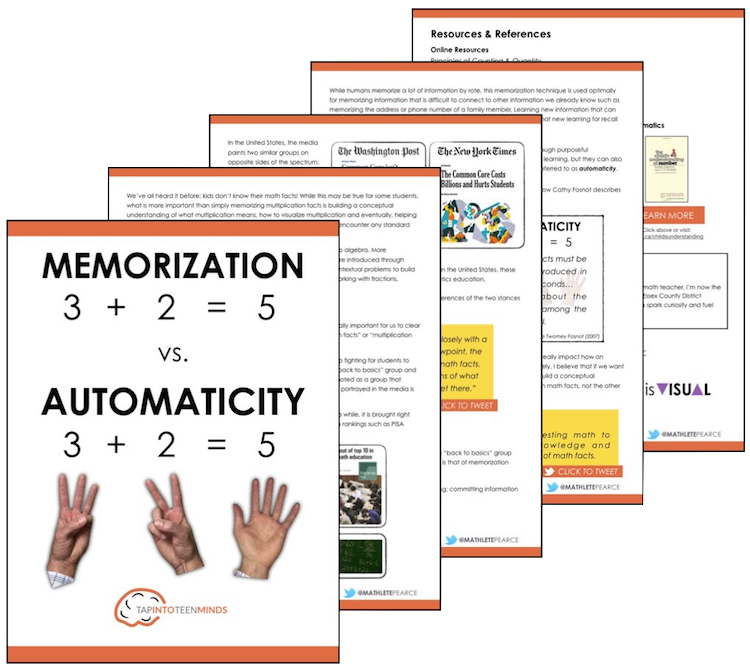## Share With Your Learning Community:I’m Kyle Pearce and I am a former high school math teacher. I’m now the K-12 Mathematics Consultant with the Greater Essex County District School Board, where I uncover creative ways to spark curiosity and fuel sense making in mathematics. Read more.

## Not What You're Looking For?

### Search By Criteria:

Spark Curiosity!